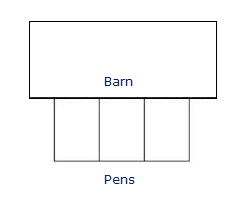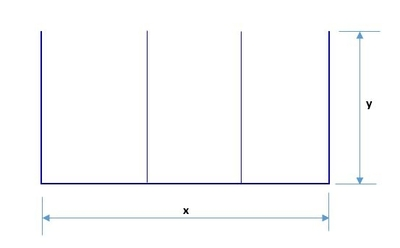# A farmer decides to make three identical pens with 88 feet of fence. The pens will be next to...

## Question:

A farmer decides to make three identical pens with {eq}88 {/eq} feet of fence. The pens will be next to each other sharing a fence and will be up against a barn. The barn side needs no fence.

What dimensions for the total enclosure (rectangle including all pens) will make the area as large as possible?## Application of Maxima and Minima:

Here, to determine the value of the quantity we will be using derivative where we will se the first derivative of the function to zero. In setting up the function we need to use the formula for the perimeter which just the sum of all the length of the sides and also the formula for the area of rectangle which is {eq}\displaystyle A=l\times w {/eq} where {eq}l,w {/eq} are the length and width.

Become a Study.com member to unlock this answer! Create your account

Below is the figure,From the figure the perimeter of the pens is,

{eq}\displaystyle P=x+4y {/eq}

{eq}\displaystyle 88=x+4y {/eq}

{e...Differential Calculus: Definition & Applications

from

Chapter 13 / Lesson 6
13K

This lesson is an introduction to differential calculus, the branch of mathematics that is concerned with rates of change. If you ever wanted to know how things change over time, then this is the place to start!